Author: 上海交通大学密码与计算机安全实验室软件安全小组GoSSIP

# 第一题

## 0x1 分析## 0x2 巧妙的解法

• 1 – 么
• 2 – 广
• 3 – 亡
• 4 – 门
• 5 – 义
• 6 – 之
• 7 – 尸
• 8 – 弓
• 9 – 己

``````581026
``````

# 第二题

## 0x1 分析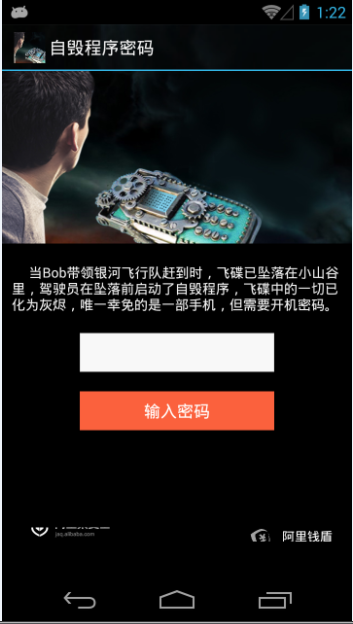## 0x2 巧妙的解法

``````I/yaotong ( XXX): SecurityCheck Started...
``````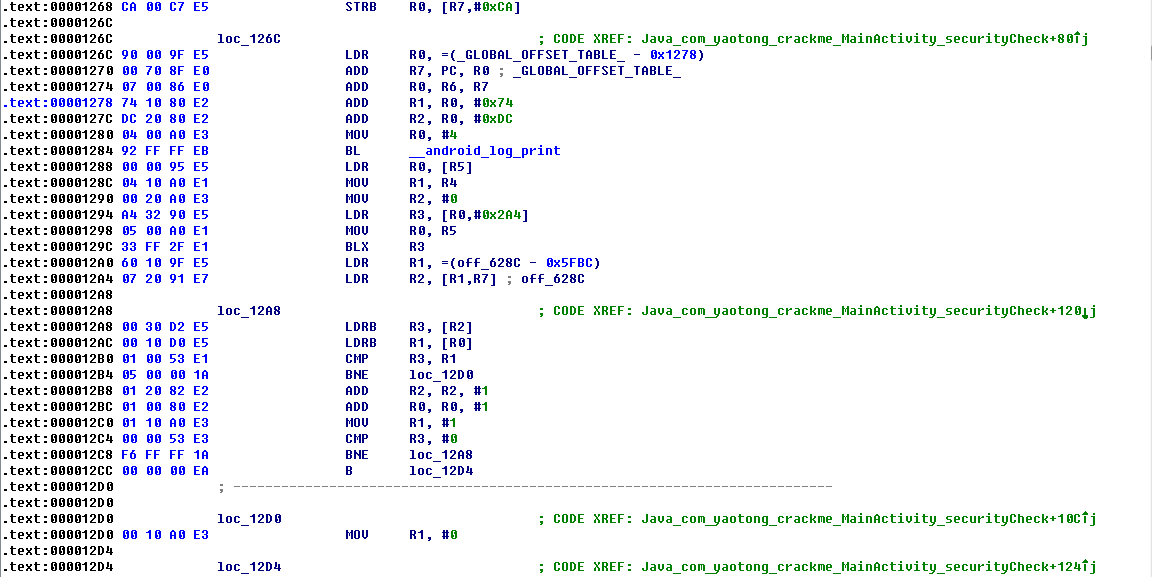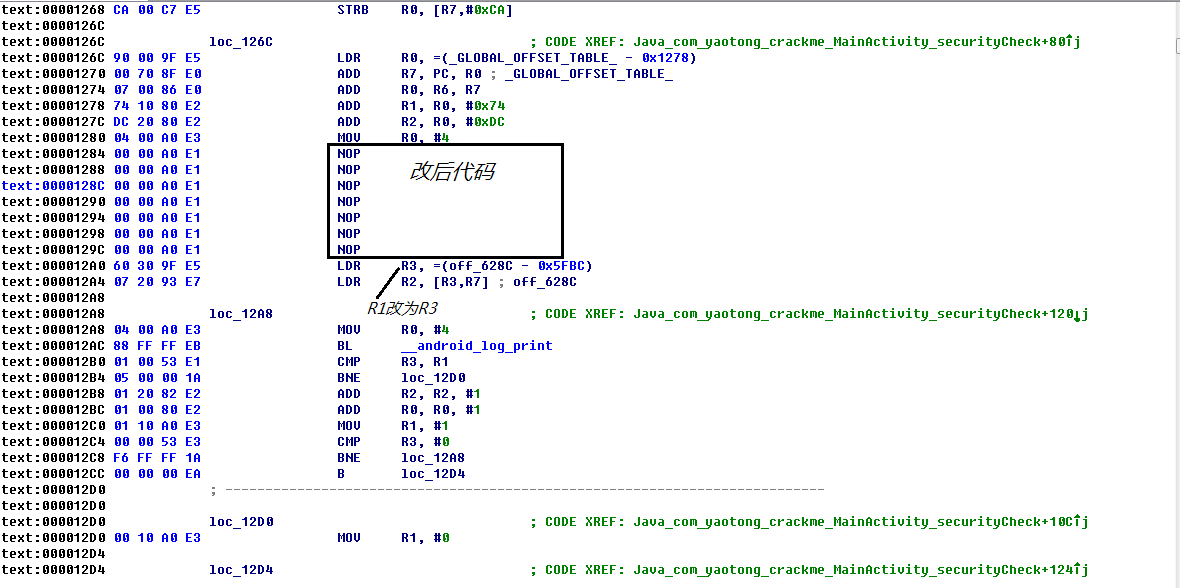``````aiyou,bucuoo
``````

# 第三题

``````#!java
for ( size_t i = 33; i < 127; ++i )
{
for ( size_t j = 33; j < 127; ++j )
{
String x =String.valueOf((char)j)+String.valueOf((char)i);
if (x.hashCode()==3618 && (i+j) == 168)
{
System.out.println(x);
System.out.println(j+i);
}
}
}
``````

``````s5
168
``````

``````// TODO: Annotations.
``````

``````s57e1p
``````

``````… _____ ____. . ..___ .__.
``````

# 第四题Dex整体处理过程和上一题也类似，使用`handleMessage`处理最后的判断输入成功与否，只有`sendEmptyMessage(0)`后，触发除以0的异常才能成功。不过这一题将用户输入转成byte后，传给一个native的方法：`ali\$a``M\$j`方法，另外参数还包括一个常数48和Handler。看样子逆向native库势在必行了。这一题的lib文件夹下文件和上一题是一样的，有三个文件，其中`libmobisecy.so`其实是个zip文件，解压后是个classes.dex，直接反汇编后，类和方法的名字都在，只是里面的代码都是

``````throw new RuntimeException();
``````

`libmobisecz.so`直接就是一堆binary数据，猜测应该是运行时会被解密，通过某种方式映射到为真正的代码执行。因此我们的目标就是`libmobisec.so`这个ELF文件。

``````[email protected]/* <![CDATA[ */!function(t,e,r,n,c,a,p){try{t=document.currentScript||function(){for(t=document.getElementsByTagName('script'),e=t.length;e--;)if(t[e].getAttribute('data-cfhash'))return t[e]}();if(t&#038;&#038;(c=t.previousSibling)){p=t.parentNode;if(a=c.getAttribute('data-cfemail')){for(e='',r='0x'+a.substr(0,2)|0,n=2;a.length-n;n+=2)e+='%'+('0'+('0x'+a.substr(n,2)^r).toString(16)).slice(-2);p.replaceChild(document.createTextNode(decodeURIComponent(e)),c)}p.removeChild(t)}}catch(u){}}()/* ]]&gt; */:/ # ps | grep crackme.a4
u0_a73    1935  126   512204 48276 ffffffff 400dc408 S crackme.a4
[email protected]/* <![CDATA[ */!function(t,e,r,n,c,a,p){try{t=document.currentScript||function(){for(t=document.getElementsByTagName('script'),e=t.length;e--;)if(t[e].getAttribute('data-cfhash'))return t[e]}();if(t&#038;&#038;(c=t.previousSibling)){p=t.parentNode;if(a=c.getAttribute('data-cfemail')){for(e='',r='0x'+a.substr(0,2)|0,n=2;a.length-n;n+=2)e+='%'+('0'+('0x'+a.substr(n,2)^r).toString(16)).slice(-2);p.replaceChild(document.createTextNode(decodeURIComponent(e)),c)}p.removeChild(t)}}catch(u){}}()/* ]]&gt; */:/ # cat /proc/1935/maps
5e0f2000-5e283000 r-xp 00000000 103:04 741132    /data/app-lib/crackme.a4-1/libmobisec.so
5e283000-5e466000 r-xp 00000000 00:00 0
5e466000-5e467000 rwxp 00000000 00:00 0
5e467000-5e479000 rw-p 00000000 00:00 0
5e479000-5e490000 r-xp 00191000 103:04 741132    /data/app-lib/crackme.a4-1/libmobisec.so
5e490000-5e491000 rwxp 001a8000 103:04 741132    /data/app-lib/crackme.a4-1/libmobisec.so
5e491000-5e492000 rw-p 001a9000 103:04 741132    /data/app-lib/crackme.a4-1/libmobisec.so
5e492000-5e493000 rwxp 001aa000 103:04 741132    /data/app-lib/crackme.a4-1/libmobisec.so
5e493000-5e4c1000 rw-p 001ab000 103:04 741132    /data/app-lib/crackme.a4-1/libmobisec.so
``````

``````[email protected]/* <![CDATA[ */!function(t,e,r,n,c,a,p){try{t=document.currentScript||function(){for(t=document.getElementsByTagName('script'),e=t.length;e--;)if(t[e].getAttribute('data-cfhash'))return t[e]}();if(t&#038;&#038;(c=t.previousSibling)){p=t.parentNode;if(a=c.getAttribute('data-cfemail')){for(e='',r='0x'+a.substr(0,2)|0,n=2;a.length-n;n+=2)e+='%'+('0'+('0x'+a.substr(n,2)^r).toString(16)).slice(-2);p.replaceChild(document.createTextNode(decodeURIComponent(e)),c)}p.removeChild(t)}}catch(u){}}()/* ]]&gt; */:/ # dd if=/proc/1935/mem of=/sdcard/alimsc4 skip=1578049536 ibs=1 count=3993600
``````

dd命令使用的数字都是十进制的，skip就是`libmobisec.so`的起始地址，count是总长度。 为了让IDA还能够识别libmobisec里的libc函数，我们还需要把libc也载入到IDA中，libc就直接从system/lib里拖出来就行了。

``````adb pull /system/lib/libc.so ./
``````

``````Java_ali_00024a_M_00024j
``````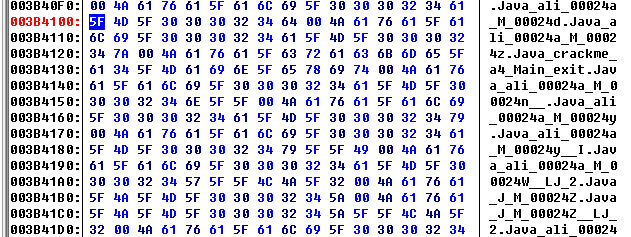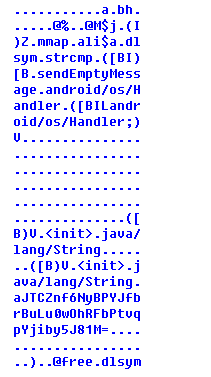``````invoke-static {}, LbKn;.a:()Z // [email protected]/* <![CDATA[ */!function(t,e,r,n,c,a,p){try{t=document.currentScript||function(){for(t=document.getElementsByTagName('script'),e=t.length;e--;)if(t[e].getAttribute('data-cfhash'))return t[e]}();if(t&#038;&#038;(c=t.previousSibling)){p=t.parentNode;if(a=c.getAttribute('data-cfemail')){for(e='',r='0x'+a.substr(0,2)|0,n=2;a.length-n;n+=2)e+='%'+('0'+('0x'+a.substr(n,2)^r).toString(16)).slice(-2);p.replaceChild(document.createTextNode(decodeURIComponent(e)),c)}p.removeChild(t)}}catch(u){}}()/* ]]&gt; */
move-result v3
invoke-static {v3}, LbKn;.b:(I)V // [email protected]/* <![CDATA[ */!function(t,e,r,n,c,a,p){try{t=document.currentScript||function(){for(t=document.getElementsByTagName('script'),e=t.length;e--;)if(t[e].getAttribute('data-cfhash'))return t[e]}();if(t&#038;&#038;(c=t.previousSibling)){p=t.parentNode;if(a=c.getAttribute('data-cfemail')){for(e='',r='0x'+a.substr(0,2)|0,n=2;a.length-n;n+=2)e+='%'+('0'+('0x'+a.substr(n,2)^r).toString(16)).slice(-2);p.replaceChild(document.createTextNode(decodeURIComponent(e)),c)}p.removeChild(t)}}catch(u){}}()/* ]]&gt; */
add-int/lit8 v0, v5, #int 1 // #01
invoke-static {v4, v5}, Lcd;.a:([BI)[B // [email protected]/* <![CDATA[ */!function(t,e,r,n,c,a,p){try{t=document.currentScript||function(){for(t=document.getElementsByTagName('script'),e=t.length;e--;)if(t[e].getAttribute('data-cfhash'))return t[e]}();if(t&#038;&#038;(c=t.previousSibling)){p=t.parentNode;if(a=c.getAttribute('data-cfemail')){for(e='',r='0x'+a.substr(0,2)|0,n=2;a.length-n;n+=2)e+='%'+('0'+('0x'+a.substr(n,2)^r).toString(16)).slice(-2);p.replaceChild(document.createTextNode(decodeURIComponent(e)),c)}p.removeChild(t)}}catch(u){}}()/* ]]&gt; */
move-result-object v1
add-int/lit8 v2, v0, #int 1 // #01
invoke-static {v1, v0}, LcC;.a:([BI)[B // [email protected]/* <![CDATA[ */!function(t,e,r,n,c,a,p){try{t=document.currentScript||function(){for(t=document.getElementsByTagName('script'),e=t.length;e--;)if(t[e].getAttribute('data-cfhash'))return t[e]}();if(t&#038;&#038;(c=t.previousSibling)){p=t.parentNode;if(a=c.getAttribute('data-cfemail')){for(e='',r='0x'+a.substr(0,2)|0,n=2;a.length-n;n+=2)e+='%'+('0'+('0x'+a.substr(n,2)^r).toString(16)).slice(-2);p.replaceChild(document.createTextNode(decodeURIComponent(e)),c)}p.removeChild(t)}}catch(u){}}()/* ]]&gt; */
move-result-object v0
add-int/lit8 v1, v2, #int -1 // #ff
invoke-static {v0, v2}, Lp;.a:([BI)[B // [email protected]/* <![CDATA[ */!function(t,e,r,n,c,a,p){try{t=document.currentScript||function(){for(t=document.getElementsByTagName('script'),e=t.length;e--;)if(t[e].getAttribute('data-cfhash'))return t[e]}();if(t&#038;&#038;(c=t.previousSibling)){p=t.parentNode;if(a=c.getAttribute('data-cfemail')){for(e='',r='0x'+a.substr(0,2)|0,n=2;a.length-n;n+=2)e+='%'+('0'+('0x'+a.substr(n,2)^r).toString(16)).slice(-2);p.replaceChild(document.createTextNode(decodeURIComponent(e)),c)}p.removeChild(t)}}catch(u){}}()/* ]]&gt; */
move-result-object v0
invoke-static {v0, v1}, Lx;.a:([BI)[B // [email protected]/* <![CDATA[ */!function(t,e,r,n,c,a,p){try{t=document.currentScript||function(){for(t=document.getElementsByTagName('script'),e=t.length;e--;)if(t[e].getAttribute('data-cfhash'))return t[e]}();if(t&#038;&#038;(c=t.previousSibling)){p=t.parentNode;if(a=c.getAttribute('data-cfemail')){for(e='',r='0x'+a.substr(0,2)|0,n=2;a.length-n;n+=2)e+='%'+('0'+('0x'+a.substr(n,2)^r).toString(16)).slice(-2);p.replaceChild(document.createTextNode(decodeURIComponent(e)),c)}p.removeChild(t)}}catch(u){}}()/* ]]&gt; */
move-result-object v0
add-int/lit8 v2, v1, #int -1 // #ff
invoke-static {v0, v1}, Lali\$a;.M\$d:([BI)[B // [email protected]/* <![CDATA[ */!function(t,e,r,n,c,a,p){try{t=document.currentScript||function(){for(t=document.getElementsByTagName('script'),e=t.length;e--;)if(t[e].getAttribute('data-cfhash'))return t[e]}();if(t&#038;&#038;(c=t.previousSibling)){p=t.parentNode;if(a=c.getAttribute('data-cfemail')){for(e='',r='0x'+a.substr(0,2)|0,n=2;a.length-n;n+=2)e+='%'+('0'+('0x'+a.substr(n,2)^r).toString(16)).slice(-2);p.replaceChild(document.createTextNode(decodeURIComponent(e)),c)}p.removeChild(t)}}catch(u){}}()/* ]]&gt; */
move-result-object v0
add-int/lit8 v1, v2, #int 1 // #01
invoke-static {v0, v2}, LaS;.a:([BI)[B // [email protected]/* <![CDATA[ */!function(t,e,r,n,c,a,p){try{t=document.currentScript||function(){for(t=document.getElementsByTagName('script'),e=t.length;e--;)if(t[e].getAttribute('data-cfhash'))return t[e]}();if(t&#038;&#038;(c=t.previousSibling)){p=t.parentNode;if(a=c.getAttribute('data-cfemail')){for(e='',r='0x'+a.substr(0,2)|0,n=2;a.length-n;n+=2)e+='%'+('0'+('0x'+a.substr(n,2)^r).toString(16)).slice(-2);p.replaceChild(document.createTextNode(decodeURIComponent(e)),c)}p.removeChild(t)}}catch(u){}}()/* ]]&gt; */
move-result-object v0
invoke-static {v0, v1}, Lx;.a:([BI)[B // [email protected]/* <![CDATA[ */!function(t,e,r,n,c,a,p){try{t=document.currentScript||function(){for(t=document.getElementsByTagName('script'),e=t.length;e--;)if(t[e].getAttribute('data-cfhash'))return t[e]}();if(t&#038;&#038;(c=t.previousSibling)){p=t.parentNode;if(a=c.getAttribute('data-cfemail')){for(e='',r='0x'+a.substr(0,2)|0,n=2;a.length-n;n+=2)e+='%'+('0'+('0x'+a.substr(n,2)^r).toString(16)).slice(-2);p.replaceChild(document.createTextNode(decodeURIComponent(e)),c)}p.removeChild(t)}}catch(u){}}()/* ]]&gt; */
move-result-object v0
add-int/lit8 v2, v1, #int 1 // #01
invoke-static {v0, v1}, Lali\$a;.M\$z:([BI)[B // [email protected]/* <![CDATA[ */!function(t,e,r,n,c,a,p){try{t=document.currentScript||function(){for(t=document.getElementsByTagName('script'),e=t.length;e--;)if(t[e].getAttribute('data-cfhash'))return t[e]}();if(t&#038;&#038;(c=t.previousSibling)){p=t.parentNode;if(a=c.getAttribute('data-cfemail')){for(e='',r='0x'+a.substr(0,2)|0,n=2;a.length-n;n+=2)e+='%'+('0'+('0x'+a.substr(n,2)^r).toString(16)).slice(-2);p.replaceChild(document.createTextNode(decodeURIComponent(e)),c)}p.removeChild(t)}}catch(u){}}()/* ]]&gt; */
move-result-object v0
add-int/lit8 v1, v2, #int 1 // #01
invoke-static {v0, v2}, Lcd;.a:([BI)[B // [email protected]/* <![CDATA[ */!function(t,e,r,n,c,a,p){try{t=document.currentScript||function(){for(t=document.getElementsByTagName('script'),e=t.length;e--;)if(t[e].getAttribute('data-cfhash'))return t[e]}();if(t&#038;&#038;(c=t.previousSibling)){p=t.parentNode;if(a=c.getAttribute('data-cfemail')){for(e='',r='0x'+a.substr(0,2)|0,n=2;a.length-n;n+=2)e+='%'+('0'+('0x'+a.substr(n,2)^r).toString(16)).slice(-2);p.replaceChild(document.createTextNode(decodeURIComponent(e)),c)}p.removeChild(t)}}catch(u){}}()/* ]]&gt; */
move-result-object v0
add-int/lit8 v2, v1, #int 1 // #01
invoke-static {v0, v1}, LcC;.a:([BI)[B // [email protected]/* <![CDATA[ */!function(t,e,r,n,c,a,p){try{t=document.currentScript||function(){for(t=document.getElementsByTagName('script'),e=t.length;e--;)if(t[e].getAttribute('data-cfhash'))return t[e]}();if(t&#038;&#038;(c=t.previousSibling)){p=t.parentNode;if(a=c.getAttribute('data-cfemail')){for(e='',r='0x'+a.substr(0,2)|0,n=2;a.length-n;n+=2)e+='%'+('0'+('0x'+a.substr(n,2)^r).toString(16)).slice(-2);p.replaceChild(document.createTextNode(decodeURIComponent(e)),c)}p.removeChild(t)}}catch(u){}}()/* ]]&gt; */
move-result-object v0
return-object v0
``````

``````aJTCZnf6NyBPYJfbrBuLu0wOhRFbPtvqpYjiby5J81M=
``````

``````#!python
#!/usr/bin/env python
# encoding: utf-8

from Crypto.Cipher import AES

def Lcda(s):
return ''.join(map(lambda x: chr((ord(x) + 3) & 0xff), s))
def de_Lcda(s):
return ''.join(map(lambda x: chr((ord(x) - 3) & 0xff), s))

def LcCa(s, a):
return ''.join([chr(((ord(s[i]) ^ a) + i) & 0xff) for i in xrange(len(s))])
def de_LcCa(s, a):
return ''.join([chr(((ord(s[i]) - i) & 0xff) ^ a) for i in xrange(len(s))])

def Lpa(s):
return s[1:] + s
def de_Lpa(s):
return s[-1] + s[:-1]

def Lxa(s):
return s.encode("base64")[:-1]
def de_Lxa(s):
return s.decode("base64")

def LaliaMd(s):
return s[:3] + chr((ord(s) - 8) & 0xff) + s[4:]
def de_LaliaMd(s):
return s[:3] + chr((ord(s) + 8) & 0xff) + s[4:]

def LaSa(s):
BS = 16
pad = lambda s: s + (BS - len(s) % BS) * chr(BS - len(s) % BS)
cc = AES.new("qqVJwt11yyLm7hVK1iI2aw==".decode("base64"), AES.MODE_ECB)
return cipher
def de_LaSa(s):
cc = AES.new("qqVJwt11yyLm7hVK1iI2aw==".decode("base64"), AES.MODE_ECB)
cipher = cc.decrypt(s)
return cipher

res = "aJTCZnf6NyBPYJfbrBuLu0wOhRFbPtvqpYjiby5J81M="

flag = de_Lcda(de_LcCa(de_Lpa(de_Lxa(de_LaliaMd(de_LaSa(de_Lxa(res))))), 49))
print flag
``````

``````alilaba2345ba
``````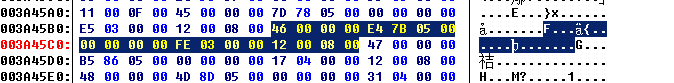# 第五题2015年移动安全挑战赛的最后一道题目，在规定的比赛时间内，仅有来自我们GoSSIP的wbyang一名选手解决了这道问题，今天我们就来揭开这一道最高难度题目的神秘面纱。dex文件并没有进行加壳和混淆，看上去是一个非常简单的程序，Java代码部分使用函数`Register("Bomb_Atlantis", input)`对输入进行判断。所以需要分析的逻辑应该都在`libcrackme.so`里的`Register`函数中。`libcrackme.so`这个动态库里使用的一些混淆方法，对于处理了前面一些类似混淆后现在的我们来说已经不是问题（^_^）。通过分析代码，我们定位了几个函数，这些函数的偏移在不同的设备上应该是不同的。整体的逻辑其实并不复杂，首先会有一个固定的字符串“Bomb_Atlantis”和一个固定的salt去进行一次md5运算，salt是动态生成的，不过由于dump内存的时候这些动态的值已经生成好了，所以能够直接发现这个salt(出于一些版权原因我们不便公布本题目的一些内部细节，因此该salt值请大家自己分析）``````h->jumpt=0x60
``````

``````push{r5,r6}
``````

``````push{r4,r5}，
``````

``````3EFoAdTxepVcVtGgdVDB6AA=
``````[微信] 扫描二维码打赏[支付宝] 扫描二维码打赏